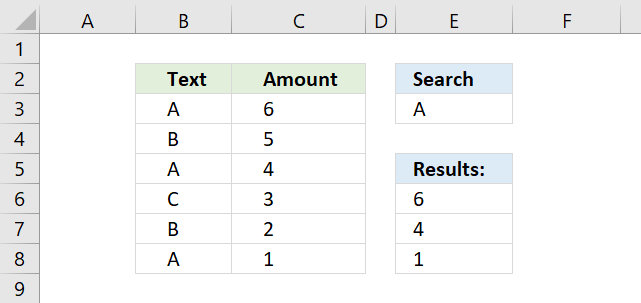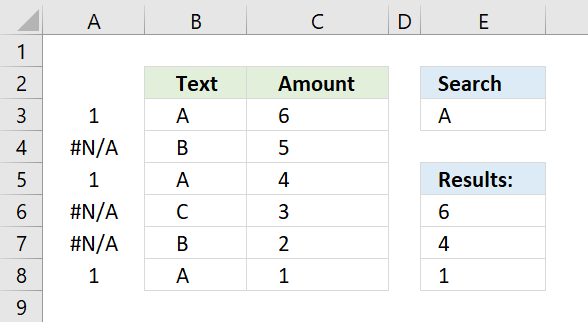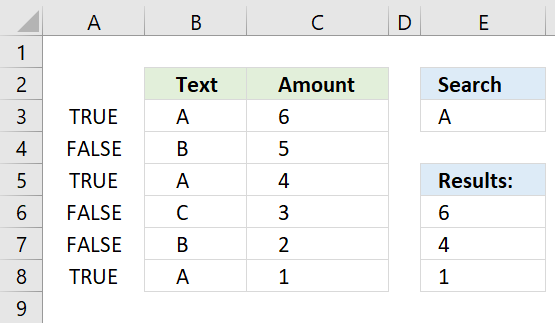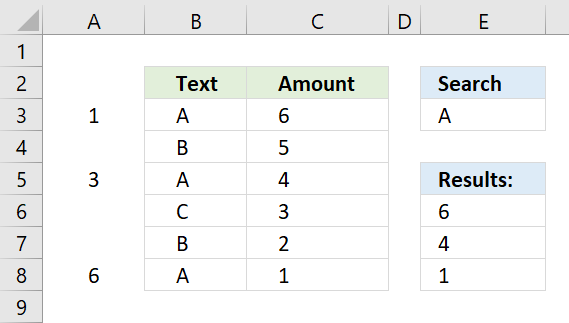Author: Oscar Cronquist Article last updated on November 08, 2021This article demonstrates how to use INDEX and MATCH functions to lookup and return multiple results. The lookup value is in cell E3, the lookup range is B3:B8.

Cells B3, B5, and B8 contains the lookup value, cell values in the corresponding cells in column C are returned. They are C3, C5, and C8.

There is actually a smaller formula that does the same thing: VLOOKUP - Return multiple values I also recommend the FILTER function if you are an Excel 365 user, the FILTER function is really easy to use.

The array formula in cell E6 extracts values from column C when the corresponding value in column B matches the value in cell E3.

The matching rows are 3, 5 and 8 so the array formula returns 3 values in cell range E6:E8.

=INDEX(\$C\$3:\$C\$8, SMALL(IF(ISNUMBER(MATCH(\$B\$3:\$B\$8, \$E\$3, 0)), MATCH(ROW(\$B\$3:\$B\$8), ROW(\$B\$3:\$B\$8)), ""), ROWS(\$A\$1:A1)))

The formula above is an array formula, make sure you follow the instructions below on how to enter an array formula to make it work.

## 1. How to enter an array formula

To enter an array formula, type the formula in a cell then press and hold CTRL + SHIFT simultaneously, now press Enter once. Release all keys.

The formula bar now shows the formula enclosed with curly brackets telling you that you entered the formula successfully. Don't enter the curly brackets yourself.

Now copy cell E6 and paste to cells below as far as needed.

## 2. Explaining formula in cell E6

#### Step 1 - Find matching values

The MATCH function matches a cell range against a single value returning an array.

MATCH(\$B\$3:\$B\$8, \$E\$3, 0)

becomes

MATCH({"A"; "B"; "A"; "C"; "B"; "A"}, "A", 0)

and returns

{1; #N/A; 1; #N/A; #N/A; 1}.

If a value is equal to the search value MATCH function returns 1. If it is not equal the MATCH function returns #N/A.The picture above displays the array in column A.

#### Step 2 - Convert array values to boolean values

The IF function cant process error values so to solve that I am going to use the ISNUMBER function to convert the array values to boolean values.

ISNUMBER(MATCH(\$B\$3:\$B\$8, \$E\$3, 0))

becomes

ISNUMBER({1; #N/A; 1; #N/A; #N/A; 1})

and returns

{TRUE; FALSE; TRUE; FALSE; FALSE; TRUE}.

The array is shown in column A, see picture below.#### Step 3 - Identify rows

The IF function converts the boolean values into row numbers and blanks.

IF(ISNUMBER(MATCH(\$B\$3:\$B\$8, \$E\$3, 0)), MATCH(ROW(\$B\$3:\$B\$8), ROW(\$B\$3:\$B\$8)), "")

becomes

IF({TRUE; FALSE; TRUE; FALSE; FALSE; TRUE}, MATCH(ROW(\$B\$3:\$B\$8), ROW(\$B\$3:\$B\$8)), "")

The MATCH and ROW functions calculate an array with sequential numbers, 1 to n, determined by the size of the cell range. In this case, \$B\$3:\$B\$8 has 6 values so the array becomes 1 to 6.

IF({TRUE; FALSE; TRUE; FALSE; FALSE; TRUE}, {1; 2; 3 ;4; 5; 6}, "")

and returns

{1;"";3;"";"";6}.

The picture below shows the relative row numbers for cell range B3:B8.#### Step 4 - Get the k-th smallest row number

To be able to return the correct value the formula must know which value to get. The SMALL function determines the value to get based on row number.

SMALL(IF(ISNUMBER(MATCH(\$B\$3:\$B\$8, \$E\$3, 0)), MATCH(ROW(\$B\$3:\$B\$8), ROW(\$B\$3:\$B\$8)), ""), ROWS(\$A\$1:A1))

becomes

SMALL({1;"";3;"";"";6}, ROWS(\$A\$1:A1))

The ROWS function returns a number that changes when you copy the cell and paste to cells below.

SMALL({1;"";3;"";"";6}, 1)

and returns 1.

In the next cell below ROWS(\$A\$1:A1) changes to ROWS(\$A\$1:A2) and returns 2.

#### Step 5 - Get values from column C using row numbers

The INDEX function returns a value from a given cell range based on a row and column number.

INDEX(\$C\$3:\$C\$8, SMALL(IF(ISNUMBER(MATCH(\$B\$3:\$B\$8, \$E\$3, 0)), MATCH(ROW(\$B\$3:\$B\$8), ROW(\$B\$3:\$B\$8)), ""), ROWS(\$A\$1:A1)))

becomes

INDEX(\$C\$3:\$C\$8, 1)

The first cell value in cell range \$C\$3:\$C\$8 is 6, the INDEX function returns 6 in cell E6.

## 3. Get Excel file

### Get the Excel fileINDEX-MATCH-multiple-resultsv2.xlsx

### Related post

Recommended articles

5 easy ways to VLOOKUP and return multiple values
This post explains how to lookup a value and return multiple values. No array formula required.